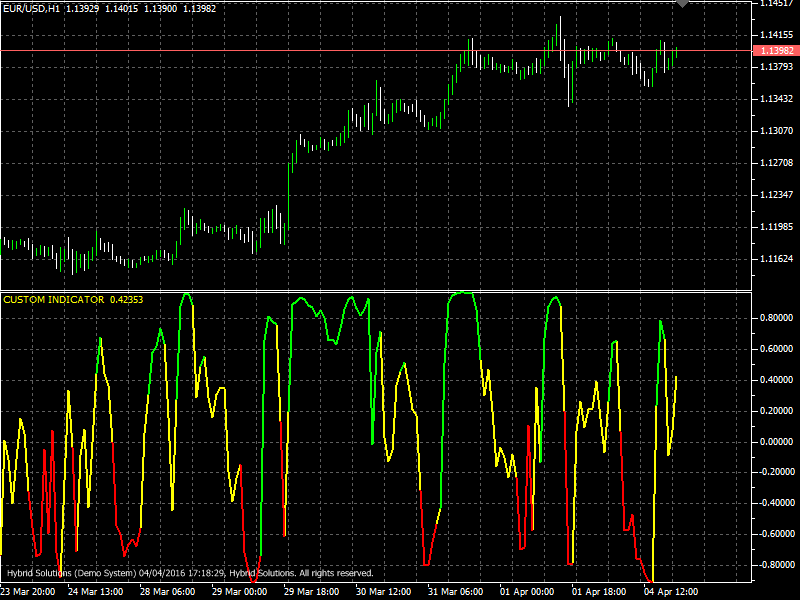# VininI RSI FO

Free

In stock

VininI RSI FO is an innovative and powerful VertexFX client side indicator that identifies market cycles and reversals in market trends, especially turning points where the market changes its direction.

SKU: 1263 Categories: ,VininI RSI FO is an innovative and powerful VertexFX client side indicator that identifies market cycles and reversals in market trends, especially turning points where the market changes its direction.

The concept behind the VininI RSI FO indicator is the method applied to calculate the Relative Strength Index (RSI). To calculate the VininI RSI FO, we first calculate the Relative Strength (RS). RS is calculated from the sum of the difference in prices over the observation period where that difference is positive (CU). Similarly, the sum of the difference in prices over the observation period where that difference is negative (CD) is calculated expressed as a positive number. RSI is calculated as 100 – 100 / (1 + CU/CD). Then we calculate the Moving Average (MA) of the Relative Strength Index over the recent MA Period bars. Next step we calculate e raised to the MA (using MathExp functions) and obtain the final value on which we build this indicator.

Overbought zone appears on the chart as a green line, and the oversold zone in the form of a red line. The yellow line is the neutral zone. This indicator generates definite buy and sell signals, as its value is close to +1 or -1 most of the time. A buy position is advised to be placed when the indicator is crossing -0.5 upwards or 0.5 upwards, in case it has crossed -0.5 upwards some time before. A sell position is adivsed when the indicator is crossing 0.5 downwards or -0.5 downwards, in case it has not crossed 0.5 downwards some time before.